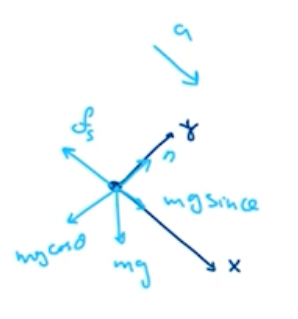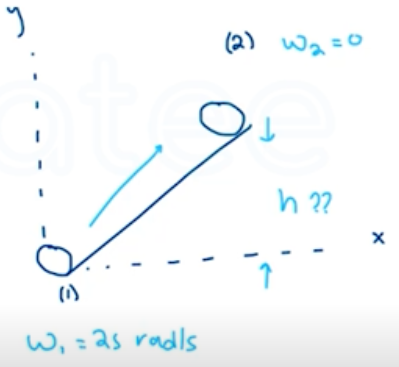Need Help?

Subscribe to Physics 1

###### \${selected_topic_name}
• Notes

$\begin{array}{l}{\text { A } 2.20-\mathrm{kg} \text { hoop } 1.20 \mathrm{m} \text { in diameter is rolling to the right }} \\ {\text { without slipping on a horizontal floor at a steady } 3.00 \mathrm{rad} / \mathrm{s} \text { . }} \\ {\text { (a) How fast is its center moving? (b) What is the total kinetic }} \\ {\text { energy of the hoop? (c) Find the velocity vector of each of the fol- }} \\ {\text { lowing points, as viewed by a person at rest on the ground: (i) the }} \\ {\text { highest point on the hoop; (ii) the lowest point on the hoop; (iii) a }}\end{array}$

$\begin{array}{l}{\text { point on the right side of the hoop, midway between the top and }} \\ {\text { the bottom. (d) Find the velocity vector for each of the points in }} \\ {\text { part ( } \mathrm{c}), \text { but this time as viewed by someone moving along with }} \\ {\text { the same velocity as the hoop. }}\end{array}$

$V_{c m}=R \omega$

$\omega=3 {rad}/ s \quad R=0.6 \mathrm{m}$

$I=M R^{2}$

$V_{c m}=(0.6)(3)=1.8 \mathrm{m} / \mathrm{s}$

$k=\frac{1}{2} M {V_{c m}}^{2}+\frac{1}{2} L \omega^{2}=\frac{1}{2} M {V_{c m}}^{2}+\frac{1}{2}\left(MR^{2}\right)\left(\frac{V_{c m}}{R^{2}}\right)$

$=M {V_{c m}}^{2}=(2.2)(1.8)^{2}=7.13 J$

$\text { (i) } V=2 V_{\text {cm }}=2(1.8)=3.6 \mathrm{m/s}$

$\text { (ii) } V=0$

$\text { (iii) } V=\sqrt{{V_{c m}}^{2}+{V_{tan}}^{2}}$

$=\sqrt{{V_{c m}}^{2}+(R \omega)^{2}}$

$=\sqrt{(1 \cdot 8)^{2}+(0 \cdot 6 * 3)^{2}}$

$=2.55 \mathrm{m/s}$

$45^{\circ} \text {below Horiz.}$

$V=V_{cm}$

$\text { (i) } V=R \omega=1.8 \mathrm{m} / \mathrm{s} \rightarrow$

$\text { (ii) } \quad V=1.8 \text { m/s } \leftarrow$

$\text { (iii) } \quad V=1.8 \text { m/s } \downarrow$

$\begin{array}{l}{\text { A solid ball is released from rest and slides down a hill- }} \\ {\text { side that slopes downward at } 65.0^{\circ} \text { from the horizontal. (a) What }} \\ {\text { minimum value must the coefficient of static friction between the }} \\ {\text { hill and ball surfaces have for no slipping to occur? (b) Would the }} \\ {\text { coefficient of friction calculated in part (a) be sufficient to prevent }} \\ {\text { a hollow ball (such as a soccer ball) from slipping? Justify your }} \\ {\text { answer.(c) In part (a), why did we use the coefficient of static fric- }} \\ {\text { tion and not the coefficient of kinetic friction? }}\end{array}$

$\sum \vec{F}_{e x t}=m \vec{a}_{c m} \quad, \quad \sum \tau_{z}=I_{cm} \alpha_{z}$$\sum F_{y}=m a_ y$

$n-m g \cos \theta=0$

$n=m g \cos \theta$

$\sum F_{x}=m a_ x \longrightarrow m g \sin \theta-f_{s}=m a$

$f_{s}=\mu_s m g \cos \theta$

$mg \sin \theta- \mu_{s} mg \cos \theta=ma \rightarrow g\left(\sin \theta-\mu_{s} \cos \theta\right)=a \rightarrow (1)$

$\sum \tau=I_{cm} \alpha_{z} \longrightarrow \tau_{f}=\mu_{s} m g \cos \theta R \ , \quad I=\frac{2}{5} m R^{2}$

$\mu_{s} m g \cos \theta R=\frac{2}{5} m R^{2} \alpha \quad \longrightarrow \alpha=\frac{a}{R}$

$\mu_{s} m g \cos \theta R=\frac{2}{5} m R^{2}\left(\frac{a}{R}\right)\rightarrow \mu_{s} g \cos \theta=\frac{2}{5} a \rightarrow (2)$

$a=\frac{5}{7} g \sin \theta \quad, \quad \mu_s=\frac{2}{7} \tan (\theta)=\frac{2}{7} \tan 65=0.613$

(b) $I=\frac{2}{3} m R^{2} \rightarrow a=\frac{3}{5} g \sin \theta$

$\mu_{s}=\frac{2}{5} \tan \theta=\frac{2}{5} \tan 65=0.858$

$\begin{array}{l}{\text { A } 392-\mathrm{N} \text { wheel comes off a moving truck and rolls with- }} \\ {\text { out slipping along a highway. At the bottom of a hill it is rotating at }} \\ {25.0 \text { rad/s. The radius of the wheel is } 0.600 \mathrm{m} \text { , and its moment of }} \\ {\text { inertia about its rotation axis is } 0.800 M R^{2} \text { . Friction does work on }} \\ {\text { the wheel as it rolls up the hill to a stop, a height } h \text { above the bot- }} \\ {\text { tom of the hill; this work has absolute value } 3500 \text { J. Calculate } h \text { . }}\end{array}$$k_{1}+U_{1}+w_{\text {other }}=k_{2}+U_{2}$

$w_{\text {other }}=W_{\text {fric }}=-3500 \mathrm{J}$

$k_{1}=\frac{1}{2} {I_{in1}}^{2}+\frac{1}{2} M{V_{1}}^{2}, V=R \omega, I=0.8 M R^{2}$

$\rightarrow k_{1}=\frac{1}{2}(0.8) M R^{2} {\omega_{1}}^{2}+ \frac{1}{2} M R^{2}{\omega_{1}}^{2}$

$=0.9 \mathrm{MR}^{2} {\omega_{1}}^{2}$

$k_{2}=0$

$U_{1}=0$

$U_{2}=m g h$

$0.9 \mathrm{MR}^{2} {\omega_{1}}^{2}-3500=\mathrm{mg} \mathrm{h}$

$M=\frac{w}{g}=\frac{392}{9.8}$

$=40 \mathrm{kg}$

$h=\frac{0.9 M R^{2} {\omega_{1}}^{2}-3500}{M g}=\frac{0.9(40)(0.6)^{2}(25)^{2}-3500}{(40)(9.8)}=11.7 m$